# Metric entropy

(diff) ← Older revision | Latest revision (diff) | Newer revision → (diff)

of a dynamical system

One of the most important invariants in ergodic theory. Basic is the concept of the entropy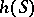of an endomorphism(see Metric isomorphism) of a Lebesgue space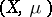. For any finite measurable decomposition (measurable partition)the limit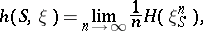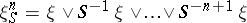(the entropy ofin unit time relative to) exists, where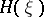is the entropy (cf. Entropy of a measurable decomposition) of, and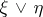is the partition whose elements are the intersections of the elements ofand. (This definition carries over verbatim towith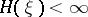; by another method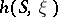can be defined for any measurable.) The entropyis defined as the least upper bound of theover all possible finite measurable. (It may be; the use of allwithor of all measurableyields the same entropy.)

Originally the entropy was defined by A.N. Kolmogorov somewhat differently (see ); the version given above came later (see ). In the basic case of an aperiodic automorphism of a Lebesgue space the definitions are ultimately equivalent .

It turns out that, and ifis an automorphism, then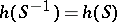. Therefore, the entropy of a cascadeis naturally taken to be. For a measurable flow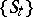it turns out that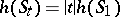. Therefore the entropy of a flow is naturally taken to be. The definition of the entropy for other transformation groups with an invariant measure is somewhat different. (It does not reduce to the entropy of a single transformation in the group; see , .) There are modifications of the entropy for the case of an infinite invariant measure ; another modification is the-entropy (where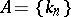is an ascending sequence of natural numbers), which is obtained whenis replaced by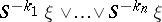and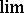by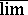(see ).

The entropy is a metric isomorphism invariant of dynamical systems and is fundamentally different from the earlier-known invariants, which are basically connected with the spectrum of a dynamical system. In particular, by means of the entropy of Bernoulli automorphisms (cf. Bernoulli automorphism; see ) it was first established that there exist non-isomorphic ergodic systems with the same continuous spectrum (which contrasts with the situation for a discrete spectrum). In a wider setting the role of the entropy is related to the fact that a new trend arose in ergodic theory: the entropy theory of dynamical systems (see , , and Ergodic theory).

The entropy provides a tool for characterizing the rate of mixing of sets of small measure (more accurately, the collection of those that form the partition). Side-by-side with this "global" role, the entropy also plays a "local" role, which is established by Breiman's ergodic theorem (an individual ergodic theorem of information theory): For ergodicand almost-all,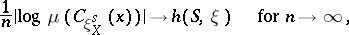where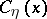is the element of the partitioncontainingand the logarithm is taken to the same base as in the definition of(see , ). (Breiman's theorem is true forwith, but, generally speaking, not for countablewith; there are variants for non-ergodic(see , ) and an infinite. A weaker assertion on the convergence in the sense of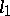has been proved for a certain general class of transformation groups .)

For smooth dynamical systems with a smooth invariant measure a connection has been established between the entropy and the Lyapunov characteristic exponent of the equations in variations (see ).

The name "entropy" is explained by the analogy between the entropy of dynamical systems and that in information theory and statistical physics, right up to the fact that in certain examples these entropies are the same (see, for example, , ). The analogy with statistical physics was one of the stimuli for introducing in ergodic theory (even in a not-purely metric context and for topological dynamical systems, cf. Topological dynamical system) new concepts such as "Gibbsian measures" , the "topological pressure" (an analogue to the free energy) and the "variational principle" for the latter (see the references to-system; Topological entropy).

How to Cite This Entry:
Metric entropy. Encyclopedia of Mathematics. URL: http://encyclopediaofmath.org/index.php?title=Metric_entropy&oldid=16474
This article was adapted from an original article by D.V. Anosov (originator), which appeared in Encyclopedia of Mathematics - ISBN 1402006098. See original article